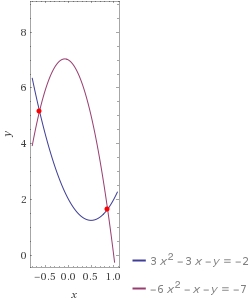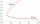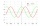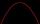# Non linear eqs

Solve the system of non-linear equations:

3x2-3x-y=-2
-6x2-x-y=-7

x1 =  0.8647
y1 =  1.649
x2 =  -0.6425
y2 =  5.1658

### Step-by-step explanation:

Our quadratic equation calculator calculates it.

${x}_{2}=\left(-0.6425\right)=-0.6425$Did you find an error or inaccuracy? Feel free to write us. Thank you!Tips to related online calculators
Looking for help with calculating roots of a quadratic equation?
Do you have a system of equations and looking for calculator system of linear equations?

## Related math problems and questions:

• If x=4If x=4 and y=-3, what is the value of the expression x² + 2xy - 4x + 3y + y²?
• Trapezoid 15Area of trapezoid is 266. What value is x if bases b1 is 2x-3, b2 is 2x+1 and height h is x+4
• EQ2Solve quadratic equation: 2x²- 2(x-7)²+5x=0
• Completing squareSolve the quadratic equation: m2=4m+20 using completing the square method
• EquationsSolve following system of equations: 6(x+7)+4(y-5)=12 2(x+y)-3(-2x+4y)=-44
• Square root 2If the square root of 3m2 +22 and -x = 0, and x=7, what is m?
• Substitution methodSolve goniometric equation: sin4 θ - 1/cos2 θ=cos2 θ - 2
• Solve equationsolve equation: [(a2)+3]1/2 + [(a2)-3]1/2 = 5
• Consider 2Consider the following formula: y = 3 ( x + 5 ) ( x - 2 ) Which of the following formulas is equivalent to this one? A. Y=3x2+9x-30 B. Y=x2+3x-10 C. Y=3x2+3x-10 D. Y=3x2+3x-30Solve pure quadratic equation -5x2 +10 = 0.
• Linsys2Solve two equations with two unknowns: 400x+120y=147.2 350x+200y=144
• Evaluate expressionEvaluate following expression: 5 to the power of 2 - (4x4) divided by 2 =
• Simple equationsSolve system of equations: 5x+3y=5 5x+7y=25
• Find the 2Find the term independent of x in the expansion of (4x3+1/2x)8
• Sphere from tree pointsEquation of sphere with three point (a,0,0), (0, a,0), (0,0, a) and center lies on plane x+y+z=a
• Intersections 3Find the intersections of the circles x2 + y2 + 6 x - 10 y + 9 = 0 and x2 + y2 + 18 x + 4 y + 21 = 0Quadratic equation 7x2+bx+c=0 has roots x1 = 67 and x2 = -84. Calculate the coefficients b and c.